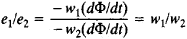Turn Ratio

The following article is from The Great Soviet Encyclopedia (1979). It might be outdated or ideologically biased.

Turn Ratio

(also turns ratio), the ratio of the electromotive forces (emf’s) induced in the primary and secondary windings of a transformer by the mutual magnetic flux, that is, the flux in the magnetic circuit. It is equal towhere e1 and e2 are the emf’s in the primary and secondary transformer windings, w1 and w2 are the numbers of turns in the primary and secondary windings, and Φ is the mutual magnetic flux. In practice the turn ratio is defined as the ratio of the rated supply voltage of the primary winding to the voltage of the open secondary winding, and the error caused by the difference between the emf and the voltage of the primary winding is neglected.

References in periodicals archive ?
Within one year of implementation, Hardin Memorial Health reduced its on hand inventory investment by more than \$500,000 and improved the inventory turn ratio from 2.6 to 7.8.
Primary SWG Secondary Turn Ratio (Np/Ns) SWG Phase X 14 [a.sub.1][a.sub.2] 1 17 [a.sub.3][a.sub.4] 0.47 17 [a.sub.5][a.sub.6] 0.47 17 Phase Y 14 [b.sub.1][b.sub.2] 0.68 17 [b.sub.3][b.sub.4] 0.858 17 [b.sub.5][b.sub.6] 0.24 17 Phase Z 14 [c.sub.1][c.sub.2] 0.68 17 [c.sub.3][c.sub.4] 0.858 17 [c.sub.5][c.sub.6] 0.24 17 Table 2.
The dc-dc converter operation is optimum only when the voltage ratio of the HVS and LVS is equal to the turn ratio of the transformer.
To prosper voltage gain ratio the coupled inductor secondary side winding are connected in series and operate as voltage source in proportion to turn ratio. Also a voltage rectifier cell composed of switched capacitor cells is embedded in the output side to achieve this target in the relative low value duty cycle.
Voltage regulation is performed by varying the transformer turn ratio. This is obtained by connecting on each phase, a tapped winding (regulation winding) in series with each 120/sqrt(3) kV winding.
Source voltage 30V Battery terminal voltage 24 V Transformer turn ratio 3:1 Inductor 13.5 [micro]H Capacitor A 40 [micro]F Capacitor B 120 [micro]F Switching frequency 50 kHz Source output resistance 10 mn Battery dynamic resistance 60 mn
A turn ratio of 5 : 2.5 : 1 for MP : FP1 : FP2 produces a turn ratio that almost matches that specified by the design procedure.
With the voltages of both sides of transformers being found out, turn ratio [N.sub.p]/[N.sub.s] can be estimated by (13)
The MC-6 has a lower rate of descent, lower opening shock, reduced canopy damage, a better turn ratio and a better glide ratio than the MC1-1C.
Marketing to a 70-80 percent turn ratio is extremely tough.
The addition of rollers enables the belts to be used for low back pressure accumulation around turns, with turning ratios of 2.8, and a tight turn ratio of 1.7.

Site: Follow: Share:
Open / Close